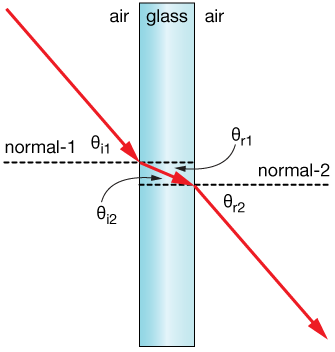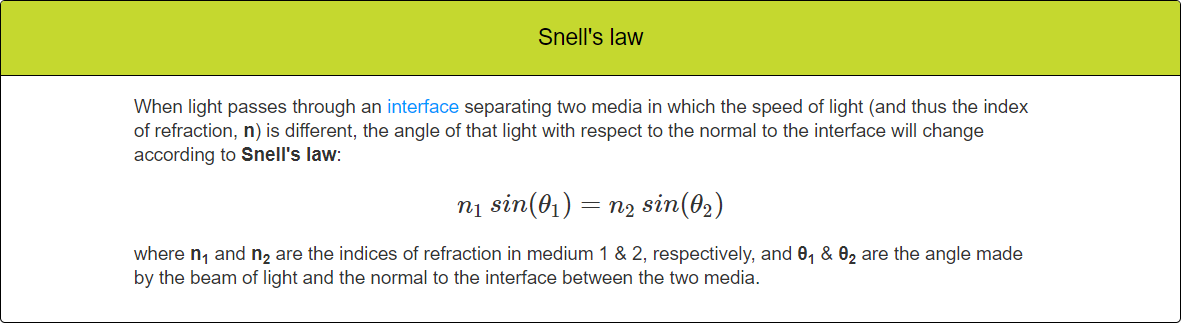Question-and-Answer Resource for the Building Energy Modeling Community
Get started with the Help page

Refraction of Light in EnergyPlus

In EnergyPlus, it is possible to find the direction of the beam solar radiation incident on a surface window.

I wondered if EnrgyPlus considers the direction of the Window Transmitted Beam Solar Radiation the same as the incident one?

In reality, the window glass has an index of refraction that causes changes in the transmitted beam solar radiation direction (due to refraction of light) when it comes from a medium with a different index of refraction such as air.

I wanted to know if EnergyPlus considers this direction change when the light passes through media with different indexes of refractions?

edit retag close merge delete

Thanks for the link. I checked it out. But I didn't find anything regarding the refraction of light.

Sort by » oldest newest most voted

For questions like this about how EnergyPlus performs calculations, you want to review the Engineering Reference documentation. There is an entire section about Window Calculations, and the index of refraction, $n$, appears in multiple places:

SUMMARY: Yes, EnergyPlus accounts for index of refraction of light and solar passing through windows.

To see the incidence angle as an output variable, your best option is likely the Surface Outside Face Beam Solar Incident Angle Cosine Value. This is from the surface object defining the fenestration (FenestrationSurface:Detailed, e.g.).

This is only for the outside face of the window -- there isn't a similar output variable for the incidence angle at the inside face of the window. However, incidence angles will only change for different materials or mediums due to their different index of refraction (air vs. glass, for example). Therefore, the incidence angle for light/solar passing through air at the outside face of the window will eventually be the same incidence angle for light/solar passing through air at the inside face of the window after transmitting through all glass layers. See image below taken from here), where $\theta_{i1} = \theta_{r2}$.more

Thank you for the answer. Yes, I checked the EnergyPlus references, and I am aware of using the index of refraction in some formulations in the EnergyPlus. However, EnergyPlus only uses the index of refraction to obtain some other radiative properties such as transmittance or reflectance. It does not use the index of refraction to consider the incidence angles changes when the light passes through different materials with different indexes of refraction. For instance, you cannot see that EnergyPlus has been used Snell's law.

Yes, I checked the EnergyPlus references, and I am aware of using the index of refraction in some formulations in the EnergyPlus.

However, EnergyPlus only uses the index of refraction to obtain some other radiative properties such as transmittance or reflectance. It does not use the index of refraction to consider the incidence angles changes when the light passes through different mediums with different indexes of refraction. For instance, you cannot see that EnergyPlus has been used Snell's law:I think, as you mentioned with the image, because the direction of light after it passes through the glass window is the same as the direction before it, EnergyPlus ignore the angle changes inside the glass layers. But, it definitely makes some errors (although small) in calculations, such as shadow calculating inside the building.

Therefore, again I emphasize my previous conclusion:

"EnergyPlus does not consider the refraction of light (incidence angles changes due to pass light through the mediums with different indexes of refraction) in calculations".

more

1